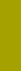﻿ Distribution VariablesSand Texture Sedimentology | Variables
DISTRIBUTION VARIABLES

The independent distribution variable (x) corresponds to the dispersity unit (Dispersitätsgrösse in German language) defined by Hans RUMPF (1975, page 8):

»Dispersity Unit is a physical quantity specified by a numerical value and unit dimension;
this quantity can describe a particle collective by a distribution function.«

In studying frequency distributions, one must be aware of meaning and suitability of both the independent and dependent variables. The independent variable is that quantity which assumes a set of values, such as grain size. The dependent variable describes the amount, frequency, of the independent variable to each of its value. We call the mathematical relationship defining the dependent variable a function of the independent variable.

In studying dispersity distributions, the most important task is choosing the appropriate independent distribution variable, because it must characterize the properties we expect to quantify, which shares the controlling dispersity property to be studied. For example, the particle surface area.

The frequency type (=dependent distribution variable, y) may have zero through three dimensions. Currently, the Macrogranometer™ system provides frequency by mass only, which is on the 3-dimensions, volume, level:

 # Frequency type Number of dimensions Suitability 1 Volume, Mass = Volume x density 3 Most common for sand-sized materials; the suitability depends on how well the physical meaning of mass (quantifies matter) is effective in a given application. In general, a mass (volume) frequency is suitable for closed sedimentation systems characterized by material conservation (the total sediment mass is constant) if no substantial material loss due to solution etc. can occur. 2 Area, grain surface, cross sectional, projected areas, etc. 2 Suitable if surface dependent physical properties (specific surface, permeability, adsorption, filtration characteristics etc.) are studied. Specific surface can be obtained from two parameters of Rosin-Rammler distribution (with mass frequency), if the mass frequency distribution of the material follows the Rosin-Rammler distribution closely (G. HERDAN, 1960, page 195) 3 Length, intercept, chord 1 Rarely used: sometime in scanning image analysis (mathematically converted into volume frequency). 4 Number, frequency by counts 0 Common in social statistics, sometime in biometrics. Only if the items occurrence is independent of their geometry, particularly size. Geometric objects such as grains can rarely justify it. Popularity of counting devices (Coulter-Counter) resulted from convenient and fast measurement. One must always be sure about the frequency type resulting from these devices. A recalculation of number frequency is possible; however, some important conditions must be met (such as a consistent and/or known grain shape, very narrow equidistant intervals of logarithmic grain size, etc.). If they are not sufficiently satisfied, the conversion errors may cause a use prohibitive.

If both the independent and dependent variables are suitably selected, the resulting distribution may closely approach the normal (Gaussian) distribution type or a mixture of several normal distributions.

The closeness of fit can be seen graphically in diagrams whose cumulative frequency on y-axis is transformed into the normal (Gaussian) distribution function, probability scale.  Its construction is shown on Fig. 1. The Gaussian cumulative frequency in linear scale (right) is projected onto a straight line of the probability scale. On Fig. 1, the integer values of the standard deviation units (σ, sigma) correspond to their Gaussian comulative percentages: 0σ corresponds to 50%, negative σ to percentages smaller than 50%, positive σ to percentages greater than 50%. Thus ±1.0σ correspond to 15.866% and 84.134% respectively, ±2.0σ correspond to 2.275% and 97.725%, ±3.0σ correspond to 0.135% and 99.865%, etc..Fig. 1: Construction of the probability scale

The following sedimentation variables can be used as independent distribution variables:

 Distribution variables: Gravity acceleration cm.sec-2 liquid temperature°C PSI — sedimentation velocity, in three versions: • laboratory measured lab terms any any any • standard calculated  standard terms 980.665 distilled water 24° • local calculated terms any*) any**) any PHI — shape-specified grain size *) Our SedVar™ can calculate gravity from latitude and altitude; **) Our SedVar™ accepts also salt water defined by temperature and salinity. grain shape — constant and PSI/PHI-dependent grain density Reynolds' number

Settling velocity distributions of monosized samples (consisting of nearly equal grain size such as narrow sieve fractions) can be converted into distributions of particle density, because the particle shape (especially for particles finer than 0.5 mm) has negligible influence on settling velocity. The density distribution reveals data for mineral identification from the density values. Our SHAPE™ program can determine the distribution components with mean(s), standard deviation(s) and percentage(s) of each component.

Our processing software SedVar also computes distributions of the grain sedimentational Reynolds' number; these distributions, similarly to those of PSI sedimentation velocity, feature a less negative skewness than the pertinent PHI grain size distributions. In fact, the skewness of the grain sedimentational Reynolds' number distributions has a medium value between the most negatively skewed PHI, and least negatively skewed PSI distributions). Not only that our Reynolds' number distributions use the shape-specified grain size but they can be computed for monosized samples.